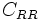# Rolling resistance

(Redirected from Rolling friction)
The magnitude of rolling resistance depends on the nature of the surfaces in contact and the magnitude of the normal force. The constant of proportionality$C_{RR}$ such that the rolling resistance is$C_{RR}$ times the normal force is termed the rolling resistance coefficient, and its magnitude is generally less than$0.01$.